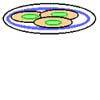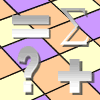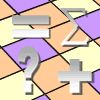Search by Topic

Resources tagged with Spreadsheets similar to Multiples Grid:

Filter by: Content type:
Age range:
Challenge level:

There are 46 resultsMultiples Grid

Age 7 to 11 Challenge Level:

What do the numbers shaded in blue on this hundred square have in common? What do you notice about the pink numbers? How about the shaded numbers in the other squares?Plates of Biscuits

Age 7 to 11 Challenge Level:

Can you rearrange the biscuits on the plates so that the three biscuits on each plate are all different and there is no plate with two biscuits the same as two biscuits on another plate?Factor-multiple Chains

Age 7 to 11 Challenge Level:

Can you see how these factor-multiple chains work? Find the chain which contains the smallest possible numbers. How about the largest possible numbers?Excel Interactive Resource: Equivalent Fraction Bars

Age 11 to 16 Challenge Level:

A simple file for the Interactive whiteboard or PC screen, demonstrating equivalent fractions.Excel Interactive Resource: Number Grid Functions

Age 11 to 16 Challenge Level:

Use Excel to investigate the effect of translations around a number grid.Excel Interactive Resource: Multiples Chain

Age 11 to 16 Challenge Level:

Use an interactive Excel spreadsheet to investigate factors and multiples.Substitution Cipher

Age 11 to 14 Challenge Level:

Find the frequency distribution for ordinary English, and use it to help you crack the code.Excel Interactive Resource: Fraction Multiplication

Age 11 to 16 Challenge Level:

Use Excel to explore multiplication of fractions.Small Change

Age 11 to 14 Challenge Level:

In how many ways can a pound (value 100 pence) be changed into some combination of 1, 2, 5, 10, 20 and 50 pence coins?Excel Interactive Resource: Haitch

Age 11 to 16 Challenge Level:

An Excel spreadsheet with an investigation.Excel Interactive Resource: Fraction Addition & Fraction Subtraction

Age 11 to 16 Challenge Level:

Use Excel to practise adding and subtracting fractions.Excel Interactive Resource: Long Multiplication

Age 11 to 16 Challenge Level:

Use an Excel spreadsheet to explore long multiplication.Excel Interactive Resource: the up and Down Game

Age 11 to 16 Challenge Level:

Use an interactive Excel spreadsheet to explore number in this exciting game!Excel Interactive Resource: Interactive Division

Age 11 to 16 Challenge Level:

Use an Excel to investigate division. Explore the relationships between the process elements using an interactive spreadsheet.Sending a Parcel

Age 11 to 14 Challenge Level:

What is the greatest volume you can get for a rectangular (cuboid) parcel if the maximum combined length and girth are 2 metres?Time of Birth

Age 11 to 14 Challenge Level:

A woman was born in a year that was a square number, lived a square number of years and died in a year that was also a square number. When was she born?What's the Weather Like?

Age 11 to 14 Challenge Level:

With access to weather station data, what interesting questions can you investigate?Cola Can

Age 11 to 14 Challenge Level:

An aluminium can contains 330 ml of cola. If the can's diameter is 6 cm what is the can's height?Litov's Mean Value Theorem

Age 11 to 14 Challenge Level:

Start with two numbers and generate a sequence where the next number is the mean of the last two numbers...Excel Investigation: Number Pyramids

Age 11 to 16 Challenge Level:

Use Excel to create some number pyramids. How are the numbers in the base line related to each other? Investigate using the spreadsheet.Excel Investigation: Pascal Multiples

Age 11 to 16 Challenge Level:

This spreadsheet highlights multiples of numbers up to 20 in Pascal's triangle. What patterns can you see?Excel Technique: Conditional Formatting

Age 11 to 16 Challenge Level:

Learn how to use conditional formatting to create attractive interactive spreadsheets in Excel.Take Ten Sticks

Age 11 to 16 Challenge Level:

Take ten sticks in heaps any way you like. Make a new heap using one from each of the heaps. By repeating that process could the arrangement 7 - 1 - 1 - 1 ever turn up, except by starting with it?Excel Technique: Triangular Arrays by Turning Off Zeros

Age 11 to 16 Challenge Level:

Learn how to use Excel to create triangular arrays.Excel Investigation: Ring on a String

Age 11 to 16 Challenge Level:

This investigation uses Excel to optimise a characteristic of interest.Excel Investigation: Happy Numbers

Age 11 to 16 Challenge Level:

Take any whole number between 1 and 999, add the squares of the digits to get a new number. Use a spreadsheet to investigate this sequence.Excel Technique: Composite Bar Charts

Age 11 to 16 Challenge Level:

Learn how to use composite bar charts in Excel.Excel Technique: Inserting an Increment Button

Age 11 to 16 Challenge Level:

Learn how to use increment buttons and scroll bars to create interactive Excel resources.Excel Technique: LOOKUP Functions

Age 11 to 16 Challenge Level:

Learn how to use lookup functions to create exciting interactive Excel spreadsheets.Excel Investigation: the Difference of Two (same) Powers

Age 11 to 16 Challenge Level:

If you take two integers and look at the difference between the square of each value, there is a nice relationship between the original numbers and that difference. Can you find the pattern using. . . .Age 11 to 16 Challenge Level:

A heap of beads was shared out by a professional bead sharer. Use the information given to find out how many beads there were at the start.Excel Technique: Making a Table for a Function of Two Independent

Age 11 to 16 Challenge Level:

Learn how to make a simple table using Excel.Excel Interactive Resource: Make a Copy

Age 11 to 16 Challenge Level:

Investigate factors and multiples using this interactive Excel spreadsheet. Use the increment buttons for experimentation and feedback.Excel Investigation: Difference Tuples

Age 11 to 16 Challenge Level:

Use an Excel spreadsheet to investigate differences between four numbers. Which set of start numbers give the longest run before becoming 0 0 0 0?Excel Investigation: Planks

Age 11 to 16 Challenge Level:

I have an unlimited supply of planks, of lengths 7 and 9 units. Putting planks end to end, what total lengths can be achieved? Use Excel to investigate.Excel Investigation: Power Crazy

Age 11 to 16 Challenge Level:

When is 7^n + 3^n a multiple of 10? Use Excel to investigate, and try to explain what you find out.Getting to Know Excel

Age 11 to 16 Challenge Level:

A number of useful techniques that can extend your use of Excel.Excel Investigation: Target Decimal

Age 11 to 16 Challenge Level:

Use an Excel spreadsheet to approximate a decimal using trial and improvement.Excel Investigation: Sums of Consecutive Numbers

Age 11 to 16 Challenge Level:Excel Investigation: Pythagorean Triples

Age 11 to 16 Challenge Level:

Use Excel to find sets of three numbers so that the sum of the squares of the first two is equal to the square of the third.Excel Investigation: Multiples of Three

Age 11 to 16 Challenge Level:

Use Excel to investigate digit sums of multiples of three. Can you explain your findings?Age 11 to 16 Challenge Level:

Use Excel to investigate remainders and divisors. Was your result predictable?Excel Technique: LCM & HCF (or GCD)

Age 11 to 16 Challenge Level:

Learn how to use the Excel functions LCM and GCD.Excel Technique: Using Paste Special to Lift a Copy of Values

Age 11 to 16 Challenge Level:Excel Technique: Logic Tests

Age 11 to 16 Challenge Level:

Learn how to use logic tests to create interactive resources using Excel.Excel Investigation: Fraction Combinations

Age 11 to 16 Challenge Level:

Choose four numbers and make two fractions. Use an Excel spreadsheet to investigate their properties. Can you generalise?